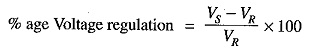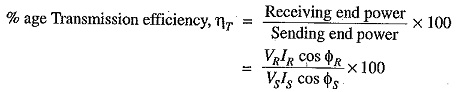## Classification of Overhead Transmission Lines:

The important considerations in the design, operation and Classification of Overhead Transmission Lines are the determination of voltage drop, line losses and efficiency of transmission. These values are greatly influenced by the line constants R,L and C of the transmission line. For instance, the voltage drop in the line depends upon the values of above three line constants. Similarly, the resistance of transmission line conductors is the most important cause of power loss in the line and determines the transmission efficiency. In this chapter, we shall develop formulas by which we can calculate voltage regulation, line losses and efficiency of transmission lines. These formulas are important for two principal reasons. Firstly, they provide an opportunity to understand the effects of the parameters of the line on bus voltages and the flow of power. Secondly, they help in developing an overall understanding of what is occurring on electric power system.

A Classification of Overhead Transmission Lines has three constants R, L and C distributed uniformly along the whole length of the line. The resistance and inductance form the series impedance. The capacitance existing between conductors for 1-phase line or from a conductor to neutral for a 3-phase line forms a shunt path throughout the length of the line. Therefore, capacitance effects introduce complications in transmission line calculations. Depending upon the manner in which capacitance is taken into account, the overhead transmission lines are classified as :

• Short transmission Lines: When the length of an overhead transmission line is upto about 50 and the line voltage is comparatively low (< 20 kV), it is usually considered as a short transmission line. Due to smaller length and lower voltage, the capacitance effects are small and hence can be neglected. Therefore, while studying the performance of a short transmission line, only resistance and inductance of the line are taken into account.
• Medium transmission lines: When the length of an overhead transmission line is about 50­-150 km and the line voltage is moderately high (>20 kV < 100 kV), it is considered as a medium transmission line. Due to sufficient length and voltage of the line, the capacitance effects are taken into account. For purposes of calculations, the distributed capacitance of the line is,divided and lumped in the form of condensers shunted across the line at one or more points.
• Long transmission lines: When the length of an overhead transmission line is more than 150 km and the line voltage is very high (> 100 kV), it is considered as a long transmission line. For the treatment of such a line, the line constants are considered uniformly distributed over the whole length of the line and rigorous methods are employed for solution.

It may be emphasized here that exact solution of any transmission line must consider the fact that the constants of the line are not lumped but are distributed uniformly throughout the length of the line.However, reasonable accuracy can be obtained by considering these constants as lumped for short and medium transmission lines.

While studying the performance of a transmission line,, it is desirable to determine its voltage regulation and transmission efficiency. We shall explain these two terms in turn.

1.Voltage regulation: When a transmission line is carrying current, there is a voltage drop in the line due to resistance and inductance of the line. The result is that receiving end voltage (VR) of the line is generally less than the sending end voltage (Vs). This voltage drop (Vs — VR) in the line is expressed as a percentage of receiving end voltage VR and is called voltage regulation.

The difference in voltage at the receiving end of a transmission line between conditions of no load and full load is called voyage regulation and is expressed as a percentage of the receiving end voltage.Obviously, it is desirable that the voltage regulation of a transmission line should be low i.e., the increase in load current should make very little difference in the receiving end voltage.

2.Transmission efficiency: The power obtained at the receiving end of a transmission line is generally less than the sending end power due to losses in the line resistance.

The ratio of receiving end power to the sending end power of a transmission line is known as the transmission efficiency of the line i.e.where VR,IR and cos ΦR are the receiving end voltage, current and power ac or while Vs, Is and cos Φs are the corresponding values at the sending end.

Scroll to Top